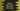# Python print current date,time,hour,minute,increment each# Python program to print current date, time, hour, minute, and increment each :

In this tutorial, we will learn how to print the current date, time and hour using python 3. Also, how to increment the day, hour or minute to the current date.

We will use the ’datetime’ module to print the current date, time, hour and minute. We will use this same module to increment the value for each.

In the example program, the time will include day, month, year, hour and minute. We will print the current time, time after one day, after four weeks, after one hour and the time after 15 minutes. Let me quickly introduce you to the python ’datetime’ module :

## datetime module :

As explained above, we are going to use the datetime module of python in this example. For that, use import datetime at the top of the program to import this module.

To get the current local date, we are using method date.today().

Again, to add one date or hour to the current date, use the timedelta() method. For example, to increment the current date-time by one hour, use timedelta(hours = 1). You can use days, seconds, microseconds, milliseconds, minutes, hours and weeks with timedelta.

The timedelta method is used to add any Delta or any time to a datetime variable.

## Python example to use datetime and timedelta :

``````import datetime

def currentTime():
print("Current date and time : ")
today = datetime.datetime.strftime(datetime.datetime.today() , '%d/%m/%Y-%Hh/%Mm')
print(today)

oneDayLater = datetime.datetime.today() + datetime.timedelta(days = 1)
currentTime()
print("Time after one day : ")
print(datetime.datetime.strftime(oneDayLater , '%d/%m/%Y-%Hh/%Mm'))

fourWeeksLater = datetime.datetime.today() + datetime.timedelta(weeks = 4)
print("")
currentTime()
print("Time after four weeks : ")
print(datetime.datetime.strftime(fourWeeksLater , '%d/%m/%Y-%Hh/%Mm'))

oneHourLater = datetime.datetime.today() + datetime.timedelta(hours = 1)
print("")
currentTime()
print("Time after one hour : ")
print(datetime.datetime.strftime(oneHourLater , '%d/%m/%Y-%Hh/%Mm'))

minutesLater = datetime.datetime.today() + datetime.timedelta(minutes = 15)
print("")
currentTime()
print("Time after 15 minutes : ")
print(datetime.datetime.strftime(minutesLater , '%d/%m/%Y-%Hh/%Mm'))``````

## Explanation :

1. We are importing the ‘datetime’ module at the start of the program. ‘import module’ is used to import a module with the name ’module’ to a python script.
2. currentTime() method is used to print the current system time. We are storing this value in the variable ’today’ and printing out the result. The current time is formatted as ‘%d/%m/%Y-%Hh/%Mm’.
3. %d directive is used to represent the day of the month as a zero-padded decimal number like 01, 02…31
4. %m directive is used to represent the month of the year as a zero-padded decimal number like 01, 02…12
5. %Y directive is used to represent the year with century as a decimal number like 0001, 0002, 2015,…9999
6. %H directive is used to represent the hour in the 24-hour format as a zero-padded decimal number like 00, 01, 02,…23
7. %M directive is used to represent the minute as a zero-padded decimal number like 00, 01,…59
8. This method is called multiple times in the program to print the current time.
9. oneDayLater variable is created by adding one day to the current time. As you can see, we are using timedelta method to create the delta time. ‘days = 1’ is passed as an argument to this method to represent that we are adding one day to the current time.
10. fourWeeksLater variable holds the time four weeks later than the current time. We are passing ‘weeks = 4’ parameter to the ’timedelta’ method.
11. Similarly, oneHourLater and minutesLater variables are holding the time one hour and 15 minutes later than the current time. We are passing ’hours = 1’ and ’minutes = 15’ as parameter to the ’timedelta’ method to create these variables. Before printing the modified time, we are calling the ’currentTime’ method to print the current time.

View on Github